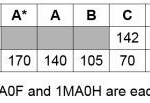:: What are the EdExcel GCSE Linear Mathematics 1MA0 May June 2016 Grade Boundaries and Mark Schemes?What are the EdExcel GCSE Linear Mathematics 1MA0 May June 2016 Grade Boundaries and Mark Scheme? Below are the EdExcel GCSE Linear Mathematics 1MA0 June 2016 Paper 1 Grade Boundaries and Mark Schemes and EdExcel GCSE Linear Mathematics 1MA0 June 2016 Paper 2

Posted in Grade Boundaries, Mark Schemes, Uncategorized

What are the EdExcel GCSE Linear Mathematics 1MA0 November 2015 Grade Boundaries and Mark Schemes?

What are the EdExcel GCSE Linear Mathematics 1MA0 November 2015 Grade Boundaries and Mark Scheme? The Grade boundaries are finally out! See below for more information. You can also check out the other grade boundaries that are available on the site.

What are the EdExcel GCSE Linear Mathematics 1MA0 November 2014 Grade Boundaries and Mark Schemes?

EdExcel GCSE Linear Maths 1MA0 November 2014 Grade Boundaries and Mark Scheme Below are the mark schemes and grade boundaries for the November 2014 EdExcel Maths GCSE exam.  Check out the previous years’ Grade boundaries and Mark Schemes to make a

Posted in Grade Boundaries, Mark Schemes, Mathematics Tagged with:

What are the EdExcel GCSE Linear Mathematics 1MA0 June 2015 Grade Boundaries and Mark Schemes?What are the EdExcel GCSE Linear Mathematics 1MA0 June 2015 Grade Boundaries and Mark Scheme? The Grade boundaries are finally out! See below for more information. You can also check out the other grade boundaries that are available on the site.

Posted in Grade Boundaries, Mark Schemes Tagged with: ,

What are the EdExcel GCSE Linear Mathematics 1MA0 June 2014 Grade Boundaries and Mark Schemes?

What are the EdExcel GCSE Linear Mathematics 1MA0 June 2014 Grade Boundaries and Mark Scheme? Below are the EdExcel GCSE Linear Mathematics 1MA0 June 2014 Paper 1 Grade Boundaries and Mark Schemes and EdExcel GCSE Linear Mathematics 1MA0 June 2014 Paper 2

What are the EdExcel GCSE Linear Mathematics 1MA0 November 2013 Grade Boundaries?

What are the EdExcel GCSE Linear Mathematics 1MA0 November 2013 Grade Boundaries? Below are the EdExcel GCSE Linear Mathematics 1MA0 November 2013 Grade Boundaries. This year’s grade boundaries is another very interesting reading especially the D to C border line on

Posted in Assessment, Grade Boundaries, Mark Schemes, Mathematics Tagged with: ,

What are the GCSE national Maths exam results figures? Useful for comparisons! [UPDATED]

What are the GCSE national Maths results figures? Below are the national GCSE figures  for Mathematics over a number of years and are very useful when making comparisons. The figures for 2013 are only preliminary and subject to change. I

Posted in Grade Boundaries, Mark Schemes, Mathematics Tagged with: ,